# Chapter3 动态规划

## 动态规划的基本思想

• 多阶段图最短路问题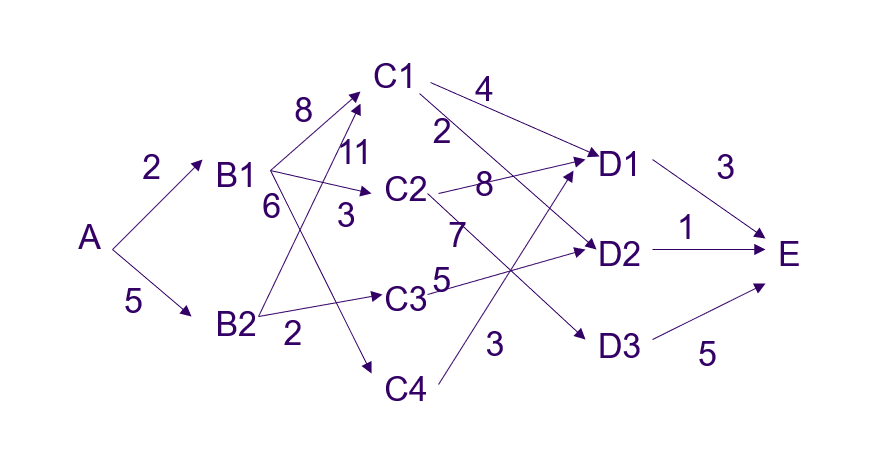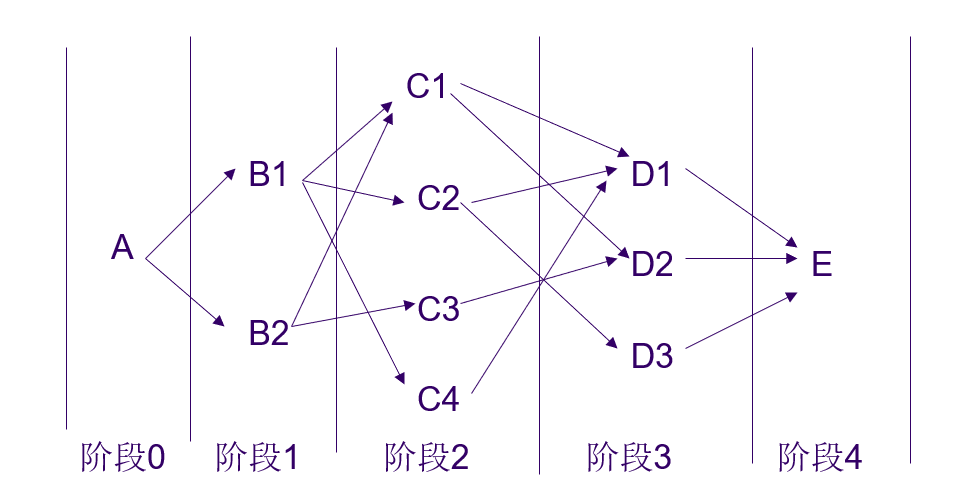• 原问题的最优解包含了子问题的最优解最优子结构

• 求解问题时

• 问题依赖的最优解

• 问题依赖的最优解

### 设计问题的步骤

• 找出最优解的性质，刻画其结构特征

• 递归的定义最优值

• 以自底向上的方式计算它

• 根据计算最优值时得到的信息，构造最优解

### 定义递归函数注意要点

• 传的参数，以及它的范围条件

• 递归的边界条件，通常在记忆化搜索中，以记录的元素存在为边界条件

 if(Memorized[i][j]) return Memorized[i][j];
• 剪枝的条件

## 矩阵连乘问题

• 如果直接顺序相乘，矩阵连乘的基本乘法数是

• 不同计算顺序的差别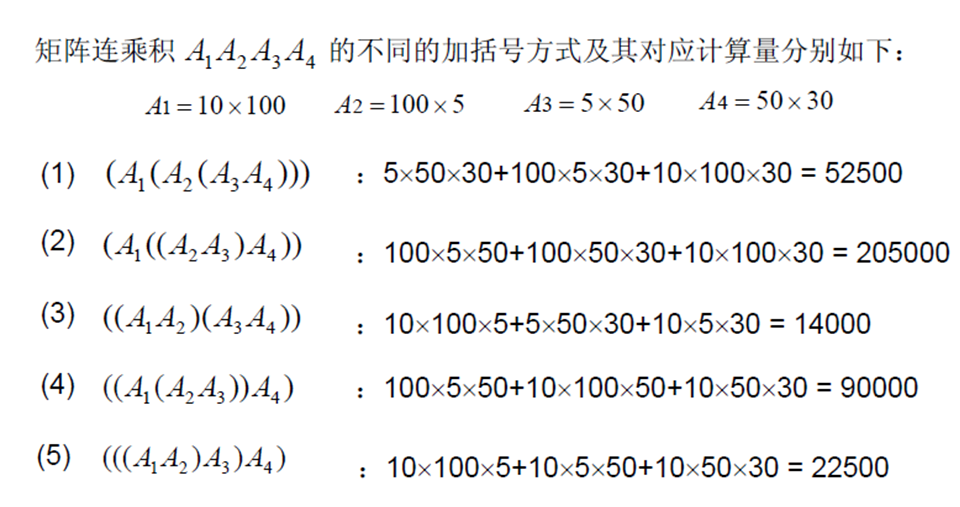• 考虑一种四个矩阵相乘的特殊情况

 int p = {p0,p1,p2,p3,p4};

• 的通用方程

设矩阵 ,是划分的位置

### 递归求解

 #include <bits/stdc++.h> ​ using namespace std; #define ll long long #define mod 1000000007 const ll maxn = 2e6 + 7; ll p[maxn]; ll m; ​ ll recursiveMatrixChain(ll i, ll j) {     if (i == j) return  m[i][j]=0;     if (m[i][j] > 0) return m[i][j];     ll minVal = recursiveMatrixChain(i, i) + recursiveMatrixChain(i + 1, j) + p[i - 1] * p[i] * p[j];     m[i][j] = minVal;     for (long long k = i ; k < j; ++k) {         ll tmp = recursiveMatrixChain(i, k) + recursiveMatrixChain(k + 1, j) + p[i - 1] * p[k] * p[j];         if (tmp < minVal) {             minVal = tmp;        }    }     m[i][j] = minVal;     return minVal; } ​ ​ int main() {     ll n;     cin >> n;     for (long long i = 0; i <= n; ++i) {         cin >> p[i];    }     int ans=recursiveMatrixChain(1, n);//注意范围，因为题目给定的n是矩阵数目-1所以是n     //如果题目了n个矩阵，那么递归的范围是[1,n-1] //   RecurMatrixChain(1,n); //   for (long long i = 0; i <= n; ++i) { //       for (long long j = 0; j <= n; ++j) { //           cout << m[i][j] << setw(5) << " "; //       } //       cout << '\n'; //   }     cout<<ans; ​     return 0; }

### 循环求解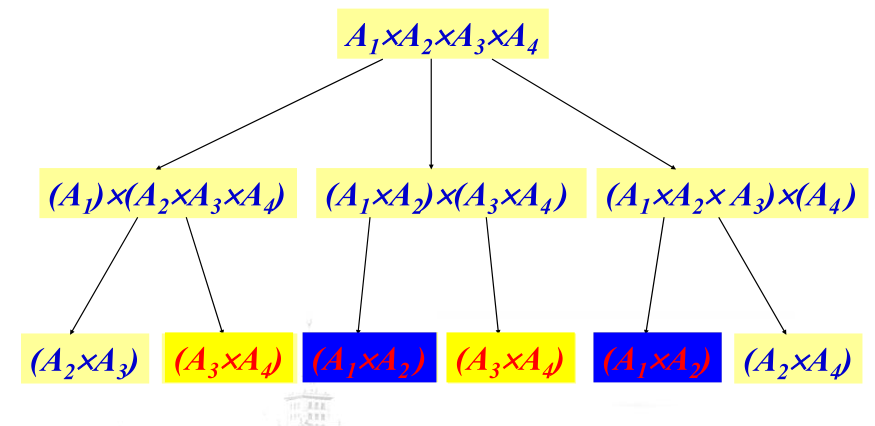• Code

 #include <bits/stdc++.h> ​ using namespace std; #define ll long long #define mod 1000000007 const ll maxn = 2e6 + 7; int m; int p; int s; int n; ​ int matrixChain() {     for (long long i = 0; i <= n; ++i) {         //对角线上的元素置0         m[i][i] = 0;    }     for (long long i = n; i >= 1; --i) {         for (long long j = i + 1; j <= n; ++j) {             //找出每一个的初值             m[i][j] = m[i][j] + m[i + 1][j] + p[i - 1] * p[i] * p[j];             s[i][j] = i;             for (long long k = i + 2; k < j; ++k) {//i+1已经比过                 //找到最小值                 int tmp = m[i][k] + m[k + 1][j] + p[i - 1] * p[k] * p[j];                 if (tmp < m[i][j]) {                     m[i][j] = tmp;                     s[i][j] = k;                }            }        }    }     return m[n]; } ​ void trace(int i, int j) {     if (i == j) {         cout << 'A' << i;         return;    }     cout << "(";     int k = s[i][j];     trace(i, k);     trace(k + 1, j);     cout << ")";     return; } ​ ​ int main() { ​     cin >> n;     for (long long i = 0; i <= n; ++i) {         cin >> p[i];    }     cout << matrixChain() << endl;     trace(1, n);     cout << endl;     for (long long i = 0; i <= n; ++i) {         for (long long j = 0; j <= n; ++j) {             cout << m[i][j] << ' ';        }         cout << '\n';    }     return 0; }

## 路径问题

### 问题集

• 数字三角形

https://www.luogu.com.cn/problem/P1216

 #include <bits/stdc++.h> ​ using namespace std; #define ll long long #define mod 1000000007 const ll maxn = 2e6 + 7; int dp; ​ int main() {     ll n;     cin >> n;     for (long long i = 0; i <= n; ++i) {         for (long long j = 0; j <= n; ++j) {             dp[i][j] = -1000000;        }    }     for (long long i = 1; i <= n; ++i) {         for (long long j = 1; j <= i; ++j) {             cin >> dp[i][j];        }    }     for (long long i = n - 1; i >= 1; --i) {         for (long long j = 1; j <= i; ++j) {             dp[i][j] += max(dp[i + 1][j], dp[i + 1][j + 1]);        }    } //   for (long long i = 0; i <= n; ++i) { //       for (long long j = 0; j <= n; ++j) { //           cout << dp[i][j] << ' '; //       } //       cout << endl; //   }     cout << dp; ​ ​     return 0; }
• 滑雪

https://www.luogu.com.cn/problem/P1434

 #include <bits/stdc++.h> ​ using namespace std; #define ll long long #define mod 1000000007 const ll maxn = 2e6 + 7; ll dx = {1, -1, 0, 0}; ll dy = {0, 0, 1, -1}; ll a; ll s; ll r, c; ​ ll dfs(ll x, ll y) {     if (s[x][y]) return s[x][y];     s[x][y] = 1;     for (long long i = 0; i < 4; ++i) {         ll xi = x + dx[i];         ll yi = y + dy[i];         if (xi > 0 && yi > 0 && xi <= r && yi <= c && a[x][y] > a[xi][yi]) {             //这里的边界有效条件除了考虑xi,yi的不能超范围，还要保证已经搜过的不能再搜的边界条件，也就是a[x][y] > a[xi][yi]             dfs(xi, yi);             s[x][y] = max(s[x][y], s[xi][yi] + 1);        }    }     return s[x][y]; } ​ int main() {     ios::sync_with_stdio(false);     cin.tie(0);     cin >> r >> c;     for (long long i = 1; i <= r; ++i) {         for (long long j = 1; j <= c; ++j) {             cin >> a[i][j];        }    }     ll ans = -1;     for (long long i = 1; i <= r; ++i) {         for (long long j = 1; j <= c; ++j) {             ans = max(ans, dfs(i, j));        }    }     cout << ans << endl; //   for (long long i = 0; i <= r; ++i) { //       for (long long j = 0; j <= c; ++j) { //           cout << s[i][j] << ' '; //       } //       cout << endl; //   }     return 0; }

## 最长公共子序列（LCS）

• LCS的结构分析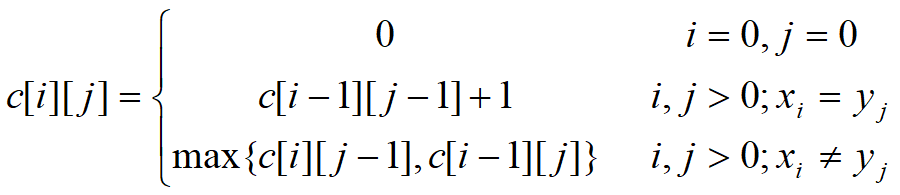• 这是一个二维表

• 填表顺序从左上角到右下角

• 时间复杂度和空间复杂度都为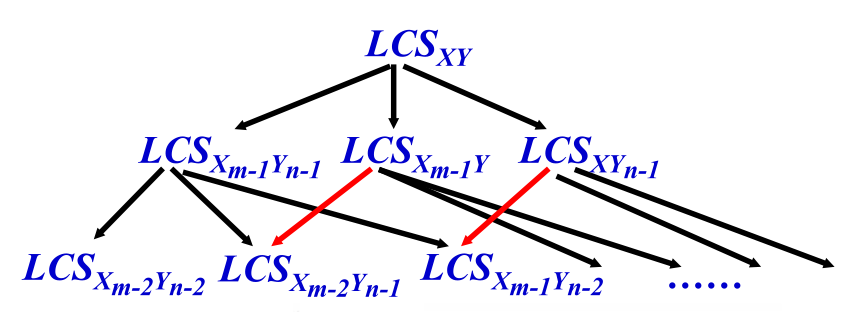• 算法思路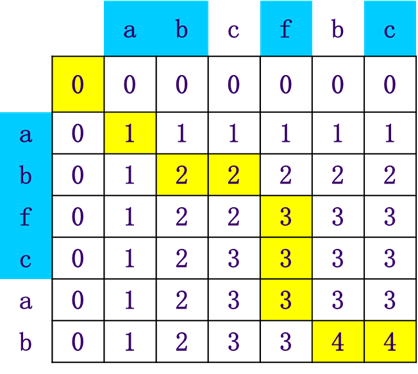• Code

 #include <bits/stdc++.h> ​ using namespace std; #define ll long long #define mod 1000000007 const ll maxn = 2e6 + 7; int c; ​ void LCSLength(char x[], char y[]) {  //调用该函数前，先将c数组置初值为0     int i, j;     for (i = 1; i <= strlen(x); i++) //自上而下         for (j = 1; j <= strlen(y); j++) { //每行自左向右             if (x[i - 1] == y[j - 1])//下标从0开始                 c[i][j] = c[i - 1][j - 1] + 1;             else if (c[i - 1][j] >= c[i][j - 1]) {                 c[i][j] = c[i - 1][j];            } else c[i][j] = c[i][j - 1];        } } ​ void LCS(int i, int j, char x[], char y[]) {     if (i == 0 || j == 0) {         return;    }     if (x[i - 1] == y[j - 1]) {//下标从0开始         LCS(i - 1, j - 1, x, y);         cout << x[i - 1];//下标从0开始    } else if (c[i - 1][j] >= c[i][j - 1]) {         LCS(i - 1, j, x, y);    } else LCS(i, j - 1, x, y); } ​ ​ char x; char y; ​ int main() {     cin >> x >> y;     LCSLength(x, y);     int lenx = strlen(x);     int leny = strlen(y); ​     cout << c[lenx][leny] << endl; ​     for (long long i = 0; i <= max(lenx, leny); ++i) {         for (long long j = 0; j <= max(lenx, leny); ++j) {             cout << c[i][j] << ' ';        }         cout << endl;    } ​     LCS(lenx, leny, x, y); ​     return 0; } /* dabcfbc eabfcab  */

posted @ 2020-11-03 15:35  幼儿算数  阅读(94)  评论(0编辑  收藏  举报